## 2.16Temperature

In the conservation of energy Eq. (2.51 ), the mechanical kinetic energy, power ﬂux and sources can be calculated from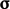,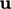and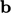from the momentum Eq. (2.19 ). Heat sources can contribute to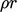, e.g. from thermal radiation, chemical reactions etc.

That leaves the heat ﬂux term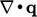which represents conduction of heat. It is commonly modelled by Fourier’s law14 which states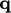is proportional to the negative gradient of temperature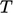, i.e.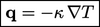(2.54)
where the constant of proportionality is thermal conductivity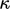.

### Temperature scale

The heat ﬂux Eq. (2.54) requires temperature to be deﬁned and measurable. Measurement requires a scale. Empirical scales correlate temperature with a measured physical property of a working substance, e.g. EMF at a junction of two metal alloys. Empirical scales have the drawbacks of: being dependent on the working substance; and, not actually deﬁning temperature.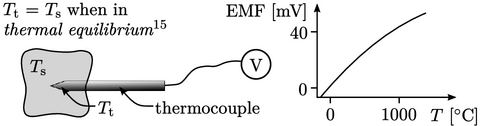Instead, the thermodynamic scale deﬁnes temperature as a measure of the average kinetic energy of random motions of particle constituents of matter. It provides an absolute measure of temperature that is independent of the choice of working substance and includes a zero point16. It must be measured in units with a zero point, such as the SI unit Kelvin,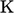.

Substitution of our model Eq. (2.54) into Eq. (2.51 ) yields the term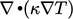. It is logical that this is a Laplacian term since it represents diﬀusion which is associated with random motions of submicroscopic particles, as we we established in Sec. 2.14 .

### Ideal gas

The behaviour of many gases under typical working conditions is captured by the ideal gas equation of state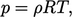(2.55)
where the speciﬁc gas constant is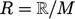. It is calculated from the Universal Gas Constant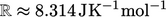in SI units, and the molar mass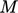of the gas, with units of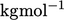.

The ideal gas equation originates from classical thermodynamics as a combination of empirical laws17. Later, it was derived from ﬁrst principles from both statistical thermodynamics and kinetic theory, with temperature representing average kinetic energy.

The derivations assume that molecules have no volume, undergo purely elastic collisions and there are no inter-molecular forces.

A scale of temperature deﬁned by the ideal gas equation of state is exactly equivalent to the thermodynamic temperature scale.

14Joseph Fourier, Théorie analytique de la chaleur, 1822.
15in accordance with the zeroth law of thermodynamics.
16in accordance with the third law of thermodynamics.
17Benoît Clapeyron, Mémoire sur la puissance motrice de la chaleur, 1834.

Notes on CFD: General Principles - 2.16 Temperature